Home Up Questions?

Chapter 8. Related Models of Parallel Computation

Petri nets have been defined as models of systems with concurrency. As we have seen, Petri nets have good modeling power, being able to model a large number of systems. However, Petri nets are not the only model of parallel computation; a number of other models have been defined, investigated, and used. In this chapter, we present some of these models and examine their relationship to Petri nets. Our purpose in this chapter is to give you a feeling for the variety of models which may be of use in modeling systems and the relative modeling power of these models.

A major problem raised by our intent to relate the various models to each other is the problem of establishing an appropriate method for comparing models of parallel computation. We wish to be able to prove that a model A is “less powerful” than a model B or is “equivalent.” The notions of equivalence and containment are of critical importance here.

Several studies have been performed on the relationships between various models. The surveys of Bredt [1970a], Baer [1973a], and Miller  helped to bring together in one place the descriptions of several models. In particular, Bredt provided a general definition of a control structure which allows the various models to be defined in a uniform way. This led to the work in [Peterson 1973] and [Peterson and Bredt 1974] which compared various models to arrive at a hierarchy of models, related by their modeling power. Independently Agerwala [1974b] compared a larger set of models and produced another hierarchy with a similar structure.

The results of both Peterson and Bredt  and Agerwala [1974b] were obtained by using the languages of the models to compare them. A class of models A defines a class of languages L ( A ) . For two classes of models A and B, the classes were defined to be equivalent if L ( A ) = L ( B ) . This means that for any specific instance a of a class of models A with language L ( a ) there would exist an instance b of class B with an identical language L ( a ) = L ( b ) . If the languages truly characterize the models, then they are an appropriate means for comparing two classes of models.

However, as we have seen, it is not completely obvious how to define a language for a model of parallel computation. Research into Petri net languages has resulted in at least 12 different definitions for languages, most of them apparently distinct. These different languages may result in different equivalence and inclusion relationships between models. On the other hand, if the differences between the models are truly significant, they may well be insensitive to (minor) variations in the definition of equivalence and inclusion. Thus, the similar results of Agerwala [1974b] and Peterson and Bredt  are more significant because of the different definitions for equivalence and inclusion used.

However, this is not to say that these results are beyond dispute. Lipton et al., also compared a large number of models of parallel computation and arrived at different results. Their comparison is based on a quite detailed analysis of the structure and state space of the specific instances of a class of models, and so their results differ significantly from those of Agerwala [1974b] and Peterson and Bredt .

So, given that there exists some difference of opinion among researchers as to how models should be compared, how shall we compare models? It would be best to take a conservative approach, and so we base our comparisons in this chapter on both structural and behavioral characteristics. We say that a class of models A is less than or equal in modeling power to (included in) a class of models B if given any instance a of the class A there is an algorithm to create an instance b of the class B such that

1. Each structural component of the model a is represented by a (small) identifiable set of components of the model b. The size of the model b ( number of parts) is at worst a constant multiple of the size of the model a, with the constant being determined by the classes of models A and B and not by the specific instances a and b.

2. Any sequence of actions in a can be simulated by a sequence in b, with the length of the sequence in b being at most a constant multiple of the length of the sequence of a.

3. The model b deadlocks only when the model a does. A model deadlocks if all actions become impossible.

The motivation for these constraints should be relatively obvious. The first constraint merely tries to establish that the two models are structurally similar; the second constraint assures that the two models behave the same. However, we do not require exactness between the two models; it is permissible to represent an action in one model by a (short) sequence of actions in another model or a component (like a place or transition) by a (small) collection of components. Thus, an action in one model can be modeled by a sequence of two actions in another model. The last constraint requires that the more powerful model cannot make a mistake when the less powerful model would not. This prevents constructing a model which nondeterministically chooses one of several actions and aborts itself if it later finds that it made the wrong choice.

Two models are equivalent if each includes the other. This allows any specific instance of either model to be converted to an instance of the other.

With this in mind we now consider the relationships among the following models of parallel computation:

1. Finite state machines [Hopcroft and Ullman 1969; Bredt 1970b; Gilbert and Chandler 1972]

2. Marked graphs [Commoner et al. 1971]

3. Computation graphs [Karp and Miller 1966]

4. P/V systems [Dijkstra 1968; Bruno et al. 1972]

5. Message systems [Riddle 1972]

6. UCLA graphs [Gostelow 1971; Cerf et al. 1971; Cerf 1972; Cerf et al. 1972]

7. Vector addition systems [Karp and Miller 1968]

8. Vector replacement systems [Keller 1972]

9. Extended Petri nets (Chapter 7)

For each class of models, we first define the model and give an example. Then we discuss its relationship to other models of parallel computation.

8.1. Finite State Machines

We have already seen in Sections 3.3.1 and 7.4.1 that finite state machines can be transformed easily into Petri nets. Finite state machines have been used by several researchers as a model of parallel computation. Bredt [1970b] defined a model based on computer hardware. Each processor is modeled as a finite state machine with input and output lines which connect a processor to other processors. The state of each input and output line is either 0 or 1. Since every output line for one processor is an input line for another processor and there are a finite number of processors and a finite number of lines each with a finite state, the entire system is finite state.

Gilbert and Chandler  used a model with common memory rather than communication lines. This means that their model is directed more toward modeling computer software processes with shared memory rather than the hardware model of Bredt but is nonetheless a finite state model and hence included in the Petri net model.

8.2. Marked Graphs

Marked graphs have been discussed in Section 7.4.2. As a subclass of Petri nets, marked graphs are obviously of more limited modeling power than Petri nets. Marked graphs are not directly comparable to finite state machines but rather seem to be duals. This provides us with the relationships in Figure 8.1 among Petri nets, finite state machines, and marked graphs.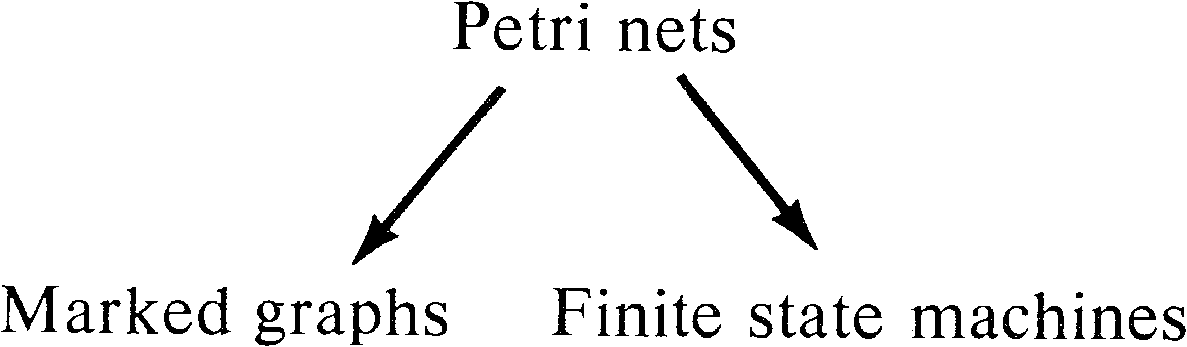Figure 8.1. Relationship of Petri nets, marked graphs, and finite state machines.

8.3. Computation Graphs

One of the earliest models of parallel computation was the computation graph model [Karp and Miller 1966]. This model was mainly designed to represent the execution of programs evaluating arithmetic expressions in parallel.

A computation graph G is defined by a directed graph G = ( V, A ), where

 V = { v1, v2, …, vn } is a set of vertices A = { a1, a2, …, am } is a set of arcs

Each arc aiA is an ordered pair of vertices ( vj, vk ) representing an arc from vj to vk. Associated with each arc ai = ( vj, vk ) is a quadruple (Ij, k, Vj, k, Wj, k, Tj, k ) . Each arc represents a queue of data items produced by the processor for node vj and to be used by the processor for node vk. Ij, k gives the number of data items initially in the queue for the arc from vj to vk. A node vk is enabled if there are at least Tj, k data items on each arc directed into node vk from each node vj; Tj, k is a threshold. The operation associated with node vk executes by removing Wj, k data items from the queue (Wj, kTj, k ) for each arc directed into vk. When the operation for vk terminates, it places Vk, r data items on the queue associated with each arc ( vk, vr ) directed from node vk to a node vr.

Figure 8.2 is an example computation graph. In the initial state, the node v1 is enabled, since it has one input and this input has three data items in its queue. When v1 executes it removes one data item from this queue and upon completion puts one data item on the arc from v1 to v2 and one on the arc from v1 to v8. In this new state either v1 or v2 can execute since both have enough data items in their input queues to satisfy their thresholds.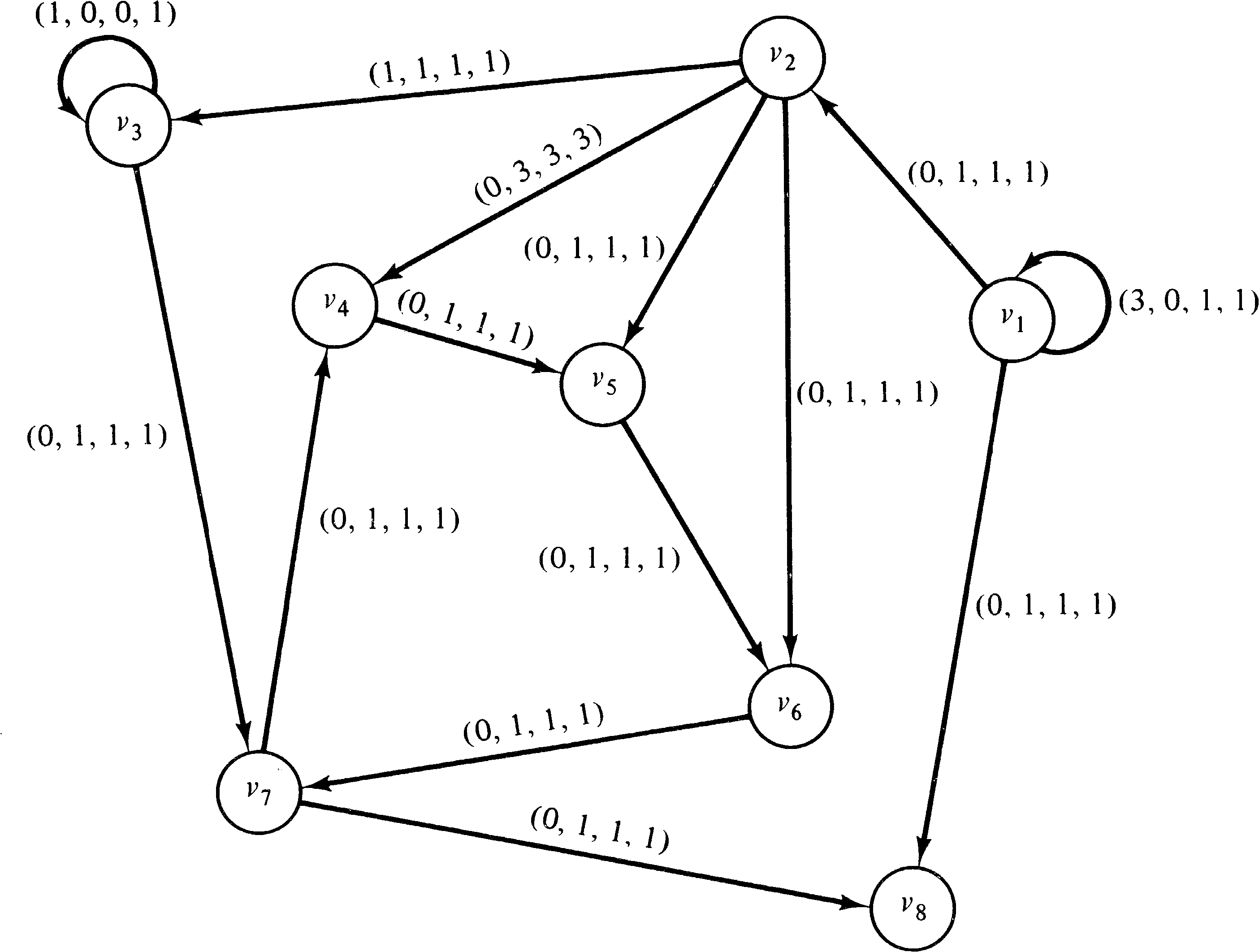Figure 8.2. A computation graph.

A computation graph is easily modeled as a Petri net. Each arc is represented by a place, and each node of the computation graph becomes a transition. The transition corresponding to node vk has Tj, k input arcs from the place representing an arc from vj to vk. This assures that the transition is enabled only if the threshold is met. However, when the transition fires it can only remove Wj, k of these tokens, so Tj, kWj, k arcs are directed back from transition vk to the place representing the arc from vj to vk. In addition Vk, r tokens are put into the places representing arcs from node vk to node vr. The initial marking is determined from the Ij, k as would be expected.

Figure 8.3 shows the Petri net constructed by this means from the computation graph of Figure 8.2.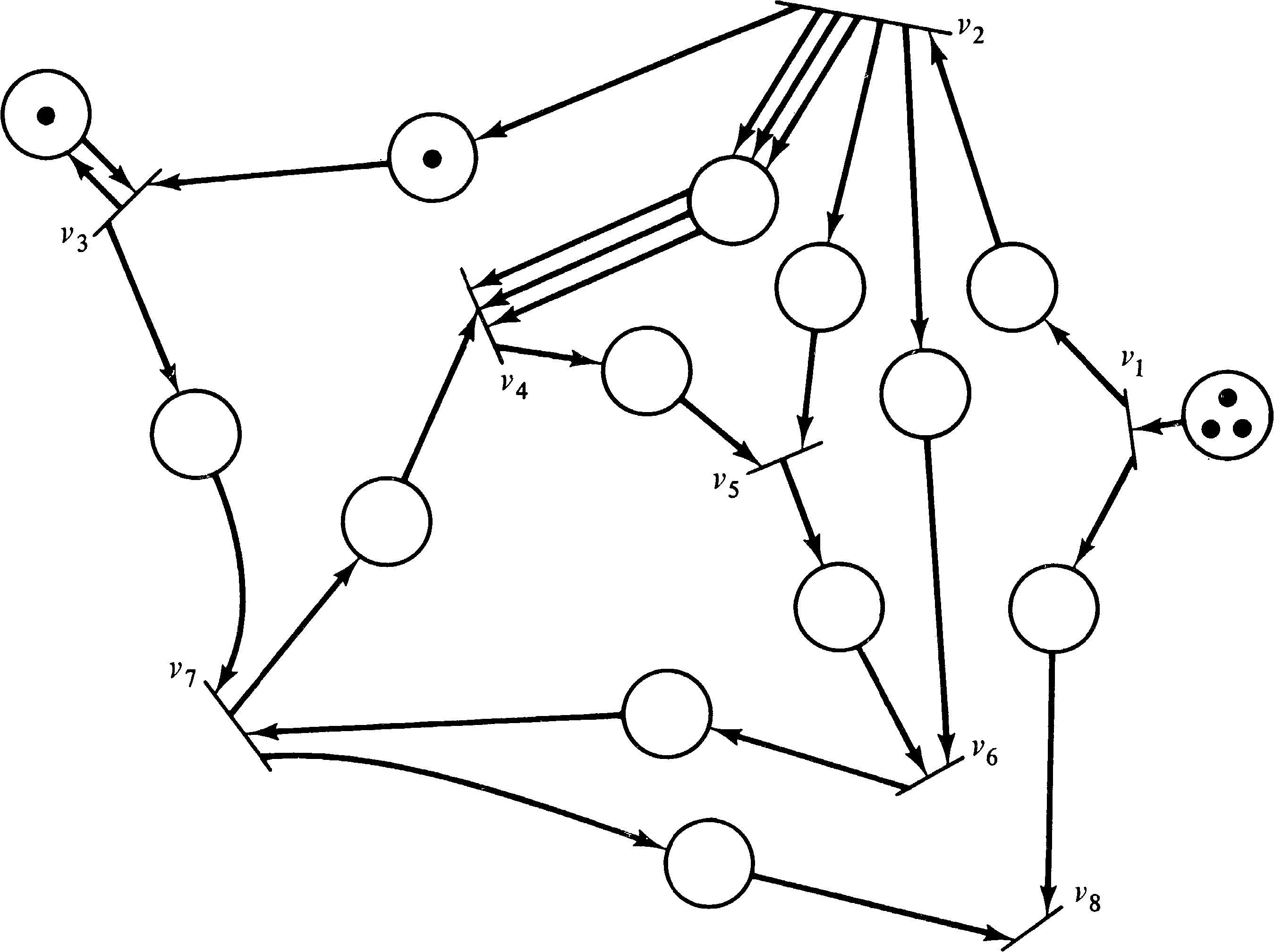Figure 8.3. A Petri net equivalent to the computation graph of Figure 8.2.

A little thought shows that marked graphs can be modeled as computation graphs with Tj, k = Wj, k for all nodes vj and vk. However, computation graphs are more powerful than marked graphs because of the ability to have Tj, k > Wj, k.

On the other hand, computation graphs and finite state machines are incomparable, as are marked graphs and finite state machines. Computation graphs cannot model decisions or conditional execution -- the same limitation that marked graphs have. Thus, our hierarchy of models at this point is as shown in Figure 8.4.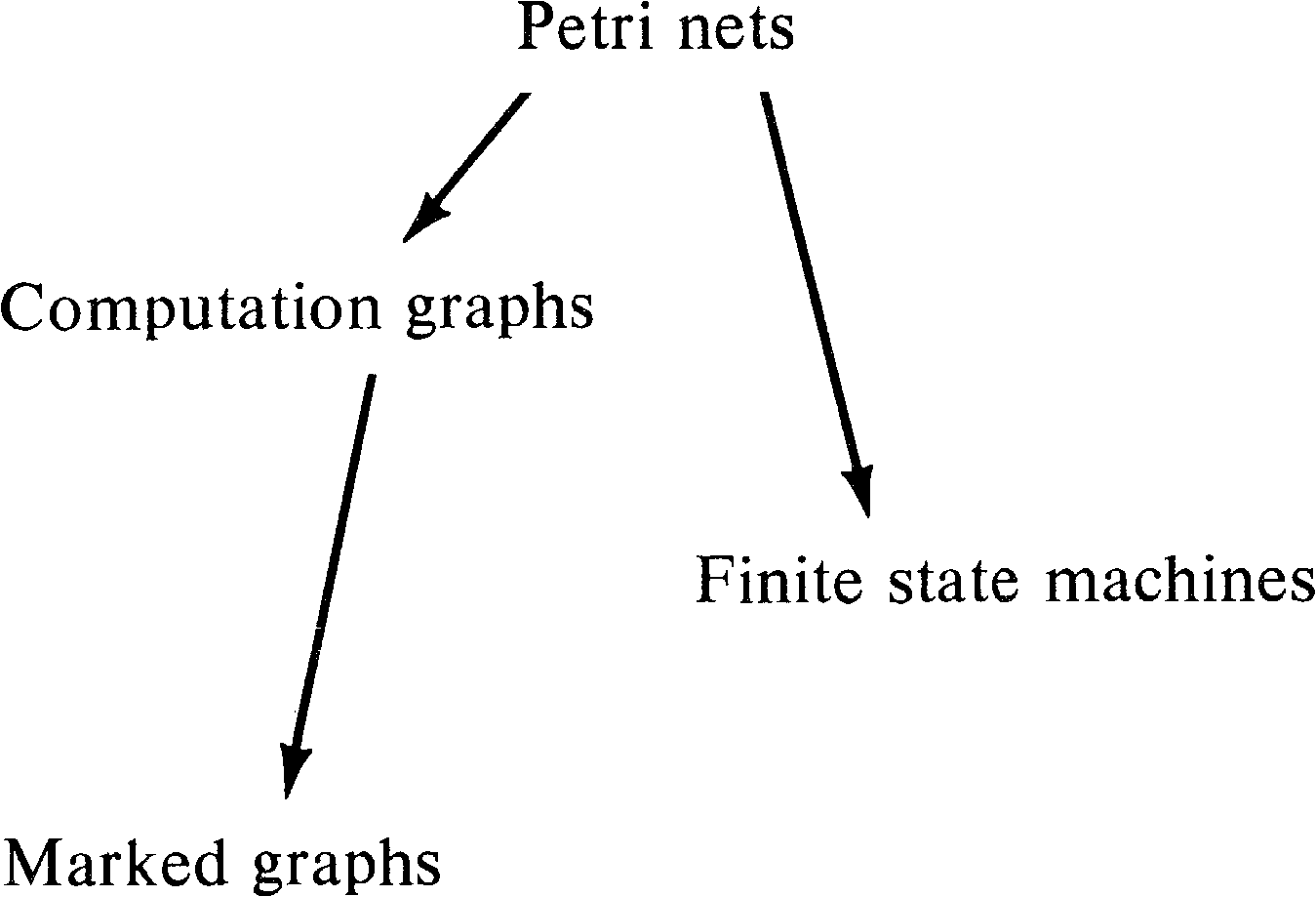Figure 8.4. Adding computation graphs to the hierarchy.

Karp and Miller  thoroughly investigated computation graphs, especially concerning the problems of liveness and safeness. Actually, Karp and Miller were interested in assuring that a computation graph would terminate and so determined the conditions for a computation graph to terminate (i.e., to not be live). Since the arcs (places) represented queues of data, Karp and Miller's investigation of boundedness was aimed at determining the maximum queue length. These differences in notation and motivation as well as the difference in model definition between computation graphs and marked graphs has meant that no one has tried to relate the results and algorithms of Karp and Miller  on computation graphs with the work on marked graphs [Commoner et al. 1971].

8.4. P/V Systems

P and V operations on semaphores were first introduced by Dijkstra  to aid in solving synchronization problems in systems of parallel processes. As such they can be used to model synchronization and communication in the same way as Petri nets. Patil used this approach when he defined the cigarette smokers' problem to show the limitations of systems which can use only P and V operations between processes. P/V systems are nonetheless quite popular, and the computing science literature abounds with discussions or applications of these operations, for example, [Liskov 1972; Brinch Hansen 1973].

Section 3.4.8 showed that P and V operations can be modeled using Petri nets, and Patil's proof [Patil 1971] shows that the inclusion is proper: There are problems (the cigarette smokers' problem, for example) which can be solved with Petri nets but not with P and V operations only. However, P/V systems are powerful enough to include both computation graph models [Lipton and Snyder 1974] and finite state machine models.

To convert a finite state machine into a P/V system, we use a separate process to model each state of the finite state machine. One semaphore is associated with each state. Let Q = { q1, q2, …, qn } be the set of states and δ: Q ⨯ Σ → Q be the next state function, with a set of actions Σ . Associate with state qi a semaphore Si and a process. The process first executes a P (Si ) . Generally it will wait here until the state of the finite state machine becomes qi. After the P (Si ), the process arbitrarily picks any σ ∈ Σ for which δ ( qi, σ ) is defined and executes a V ( Sj ), where qj = δ ( qi, σ ) . Then this process loops back to its P (Si ) . Figure 8.5 illustrates this conversion on the finite state machine of Figure 8.6. The semaphores are initially zero, except for the semaphore for the start state, which is initialized to one.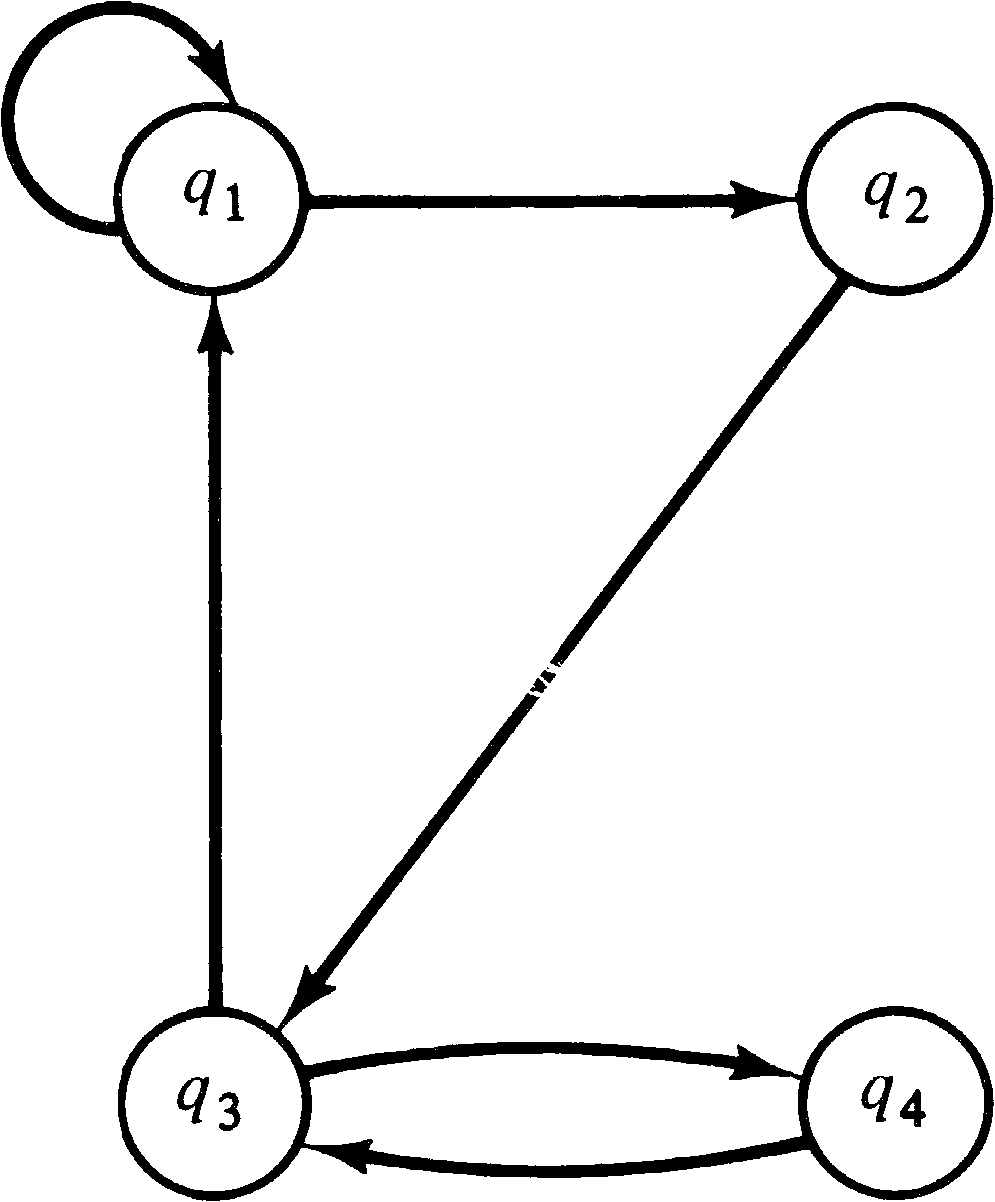Figure 8.5. A finite state machine.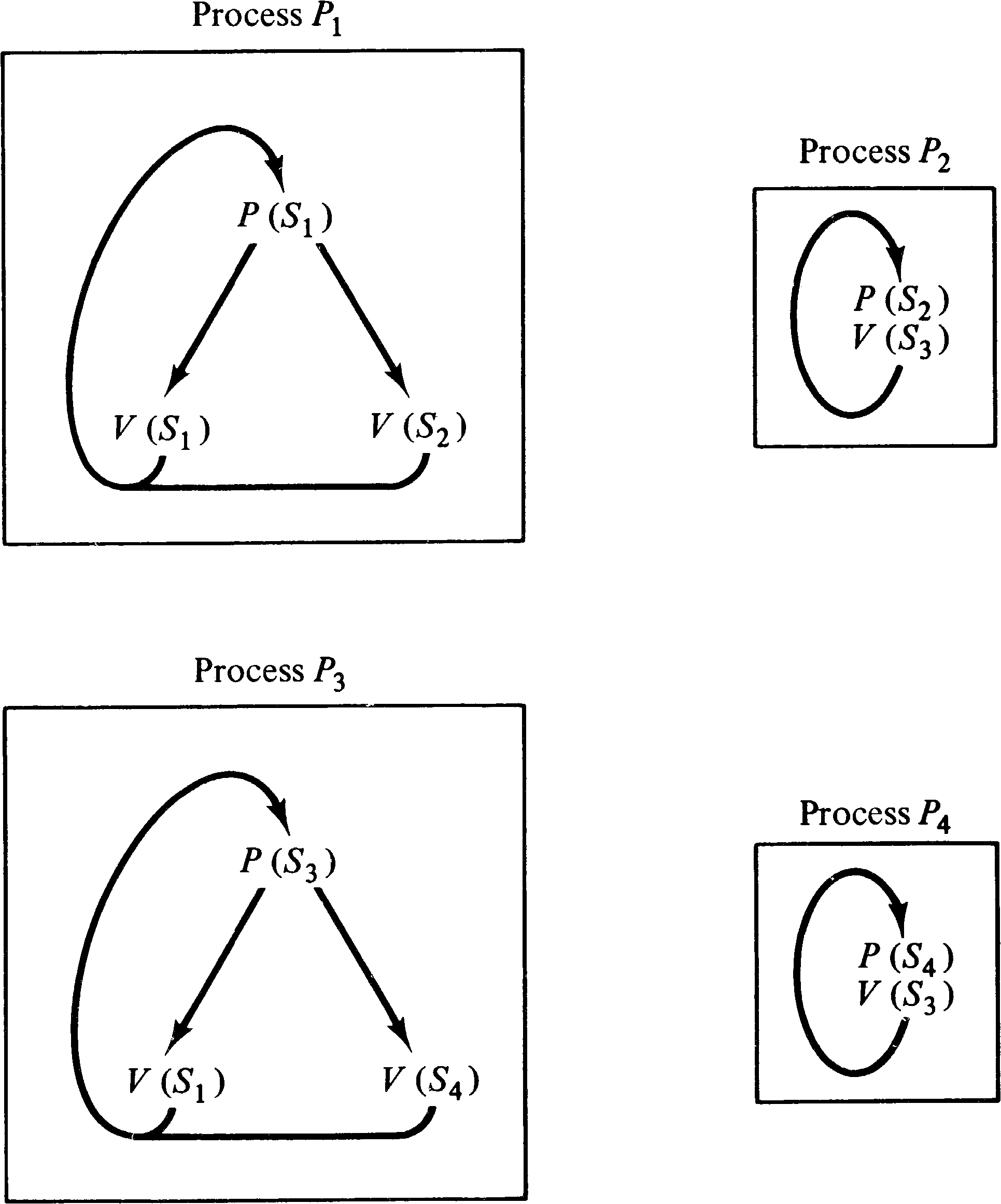Figure 8.6. P/V system for the finite state machine of Figure 8.5.

To convert a computation graph into a P/V system, we associate a semaphore Sj, k with each arc ( vj, vk ) in the graph. The value of the semaphore will be the number of data items waiting on the queue for that arc. Thus, initially the value of semaphore Sj, k is Ij, k. A process is created for every node in the computation graph. The process for node vk first executes Tj, k P operations on semaphore Sj, k for all arcs ( vj, vk ) directed into vk. This assures that each queue has at least Tj, k data items in it. Then, since each P operation decremented the semaphore and the correct effect is only to decrease Sj, k by Wj, k, we execute Tj, kWj, k V operations on Sj, k to restore the correct value of Sj, k. Now we complete the process for node vk by executing Vk, r V operations on semaphore Sk, r for each arc ( vk, vr ) directed out of node vk.

This conversion is illustrated in Figure 8.7 for the nodes v3 and v4 of the computation graph of Figure 8.2.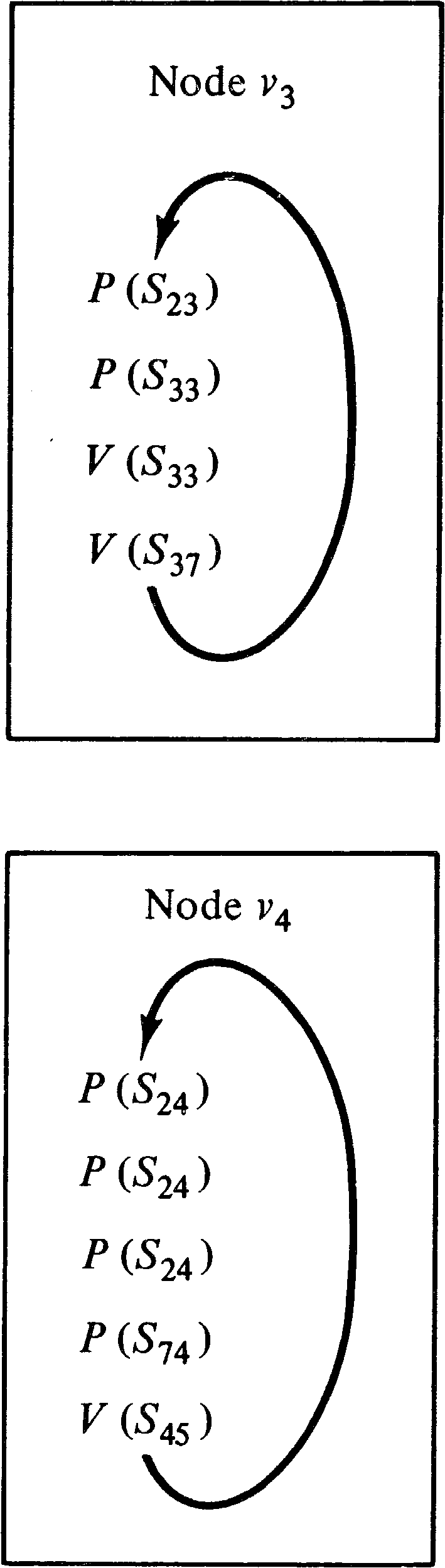Figure 8.7. P/V system processes for two of the nodes of the computation graph of Figure 8.2.

Notice that the computation graph could test and input from several sources at once, while P/V systems can only test and input from several sources by a sequence of tests and inputs from single sources. The inability to test and input from several sources simultaneously is the key to Patil's proof of the limitations of P/V systems, the problem being that another process may grab the second source while you grab the first, leading to a deadlock. This is not a problem with computation graphs because the sources are not shared between processors -- no two nodes ever share an input arc. This point is crucial to constructing a P/V system which will not deadlock (terminate) unless the corresponding computation graph also terminates.

The addition of P/V systems to our hierarchy of models gives us Figure 8.8.Figure 8.8. Adding P/V systems to the hierarchy of models.

8.5. Message Transmission Systems

Systems in which processes communicate via P and V operations on semaphores may be doing so for lack of a better communication mechanism. One suggestion for a better mechanism is messages. A message system is a collection of processes which communicate by messages. Two operations on messages are possible: send and receive. Sending a message is similar to a V operation; receiving a message is similar to a P operation. If no message is present when a receive is executed, the receiver waits until a message is sent.

This mechanism has been used as the basis of a modeling scheme by Riddle . This model would seem most appropriate for modeling computer network protocols. Riddle considers a (finite) set of processes which communicate by means of messages. Messages are sent to and requested from special processes called link processes (mailboxes). Link processes provide what is essentially a bag of messages which have been sent and not yet received or requests for messages from receives which have been made but not yet satisfied. The other processes of the system are called program processes and are represented in the program process modeling language (PPML).

An example system of three processes is given in Figure 8.9. As can be seen, the PPML description of the processes is essentially a schema. Only the transmission activity of messages in the system is of interest. Messages are abstract items whose only characteristic is a type. There may only be a finite number of types of messages in a system. Messages are sent from and received into a message buffer in each process. There is only one buffer per process. The statements in PPML areFigure 8.9. A system of Processes described in the Program Process Modeling Language (PPML).

• set t: Place a message of type t into the message buffer.

• send l: Send the message in the message buffer to link process l.

• receive l: Request a message from link process l. Wait (if necessary) until one is returned. The message is placed in the message buffer.

• unless t s: Test the type of the message in the message buffer and branch to statement s unless the message is of type t.

• if-internal-test s: Model an internal, data-dependent test. Either continue with the next statement or branch to the statement labeled s.

• go-to s: Transfer control to the statement s.

• end: Terminate the process.

The PPML system models a set of parallel processes. Each process is started at the beginning of its program and executes its program until it encounters an end statement. Riddle shows how to construct a message transfer expression which represents the possible flow of messages in the system and uses this expression to examine the structure of the system for correct operation. This message transfer expression is used for the same purpose as the language of a Petri net. Therefore, we show how a PPML description of a system of processes can be converted into a Petri net whose language is equal to the message transfer expression of Riddle's analysis. This conversion ignores the execution of the individual statements of the PPML descriptions, although with only minor modification these could also be represented in the Petri net language.

To model a process as a Petri net, we use one token per process as a program counter. The presence of a message in a link process is also represented by a token. Since the messages are identified by type, it is necessary to model each type of message in a link process by a separate place. A very important property of PPML systems is that the number of message types is finite. Each program process is also finite. Only the queueing of messages involves a potentially unbounded amount of storage. Thus the ability to model the link processes and to represent the send and receive statements correctly is the most important aspect of the transformation from a PPML description to a Petri net. By modeling the link processes by a set of places (one for each message type), we can represent a send statement by a transition which places a token in the place representing the appropriate link process and message type. The receive statement merely removes a token from any one of the places of a link process. The particular place which supplies the token determines the type of the message which was received. This information can be used in any subsequent unless statement.

The only symbols in a message transfer expression are the message types for the messages which are sent to or received from a link process. Since every transition in a Petri net results in a symbol in the Petri net language for that Petri net, only the send and receive statements in a PPML system can be modeled. Thus there are two kinds of places in the Petri net. One kind of place, labeled pli, mj, acts as a counter of the number of messages of type mj in link process li. The other kind of place represents the send and receive statements of the PPML programs. Let the statements be uniquely labeled s1, …, sr. We label the place representing a statement si with a message of type mj in the message buffer by psi, mj. A token in a place associated with a statement si means that statement si has just been executed. Figure 8.10 illustrates how a send and receive statement at sk would be modeled in the Petri net. In Figure 8.10, place psi, m represents the place associated with any statement which precedes the statement sk.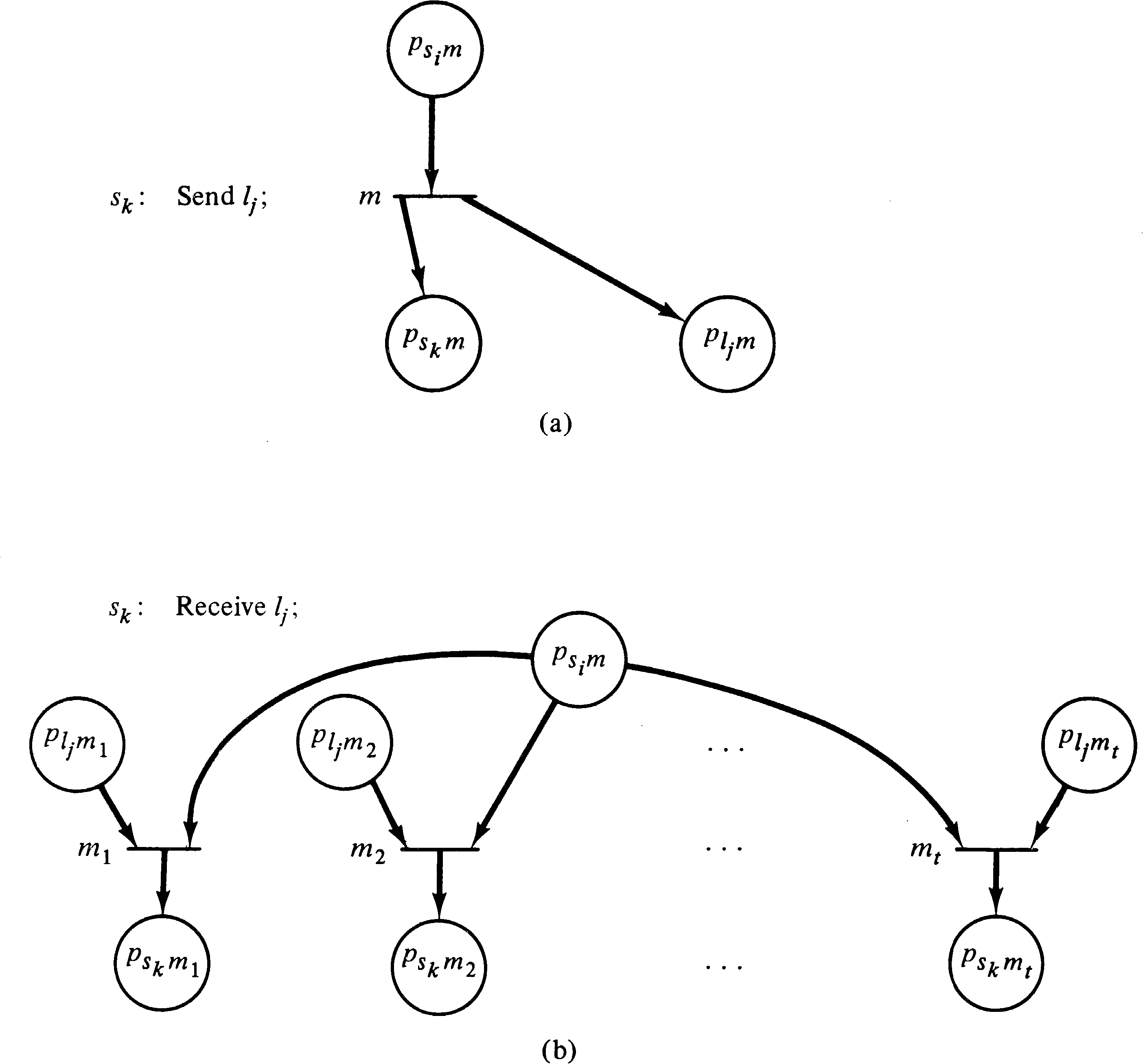Figure 8.10. Transformation from send and receive statements to Petri net transitions. (a) Model of a send statement at sk with a message of type m in the message buffer. The link process is lj. (b) Model of a receive statement at sk from link process lj. The possible message types in lj are m1, m2, …, mt.

It remains only to show that it is possible to determine which statements can precede other statements in the PPML program. Notice that we must consider each statement as a pair consisting of a message type and a statement number, since the same statement with different message types in the message buffer will be modeled differently in the Petri net. The most obvious method to determine the predecessors of a statement is to start at the beginning of each PPML program with a special start statement (which will become the start place) and follow the program description generating all possible send and receive statements which can follow this statement with their corresponding message buffer contents. This process is repeated for all newly reachable statements until all such send and receive statements have been generated and their successors identified. Since the number of statements in a PPML description and the number of message types are finite, only a finite number of statement/message-type pairs will be generated. This procedure is similar to the characteristic equations used by Riddle  to construct the message transfer expression. Figure 8.11 lists the statements and their possible successors for the PPML system of Figure 8.9.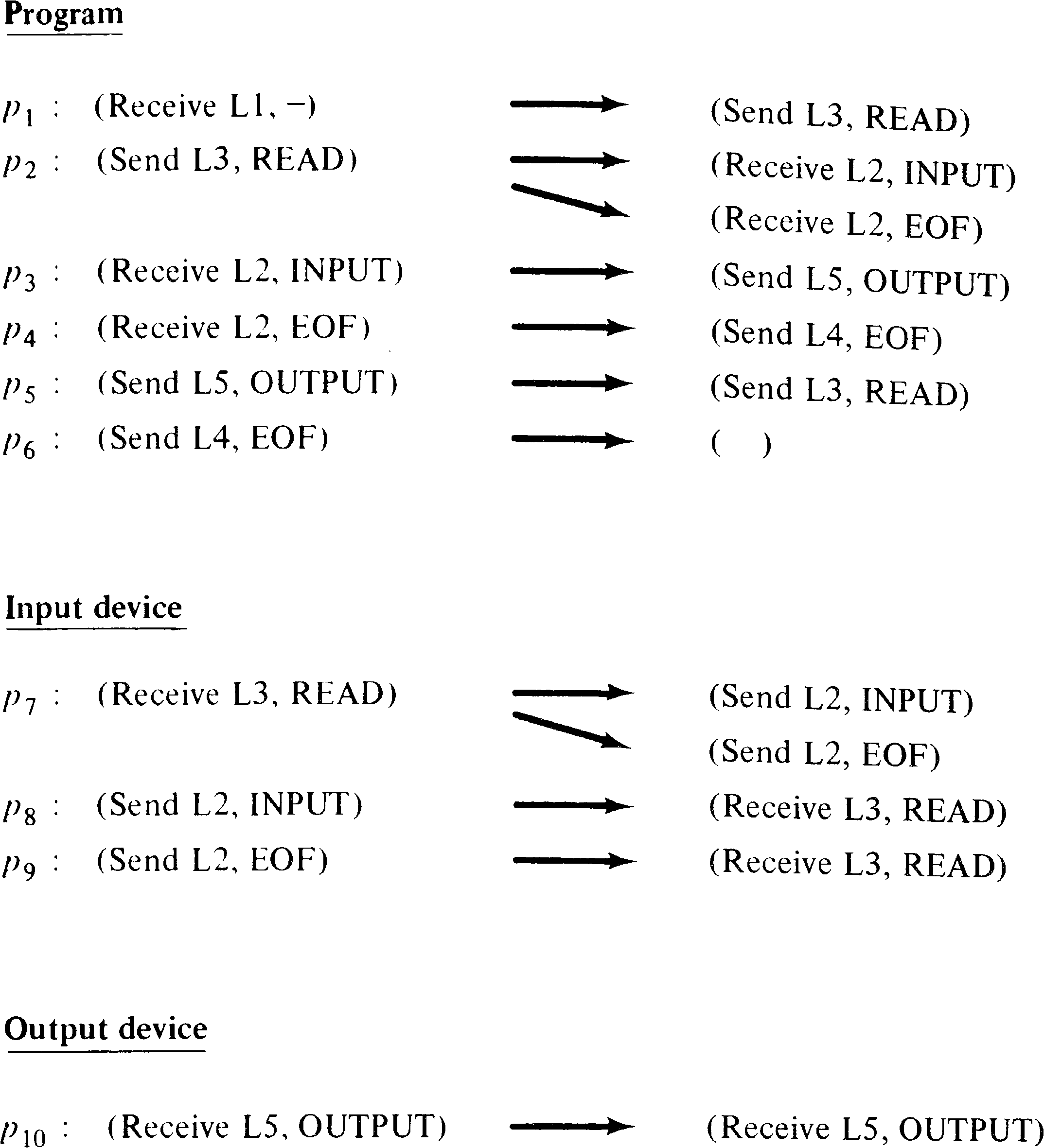Figure 8.11. Successor statements for the PPML system of Figure 8.9.

Once the successors of a statement have been determined, this information can be used to identify the possible predecessors of a statement and hence to construct a Petri net which is equivalent to the PPML system by use of transitions such as given in Figure 8.10. A special start place is the predecessor of the first statements of each of the processes of the system. Figure 8.12 transforms the PPML system of Figure 8.9 into an equivalent Petri net.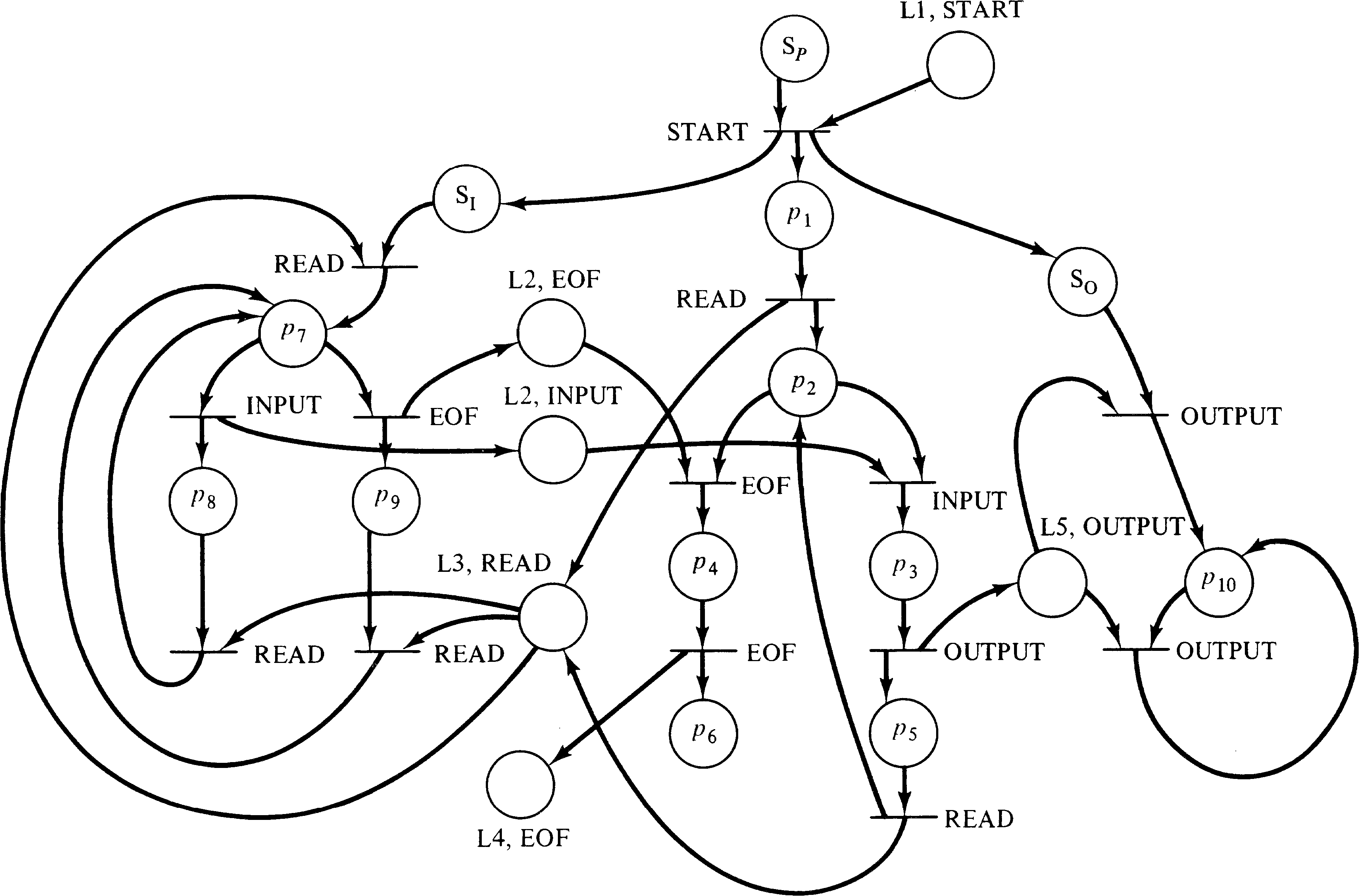Figure 8.12. A Petri net equivalent to the PPML system of Figure 8.9.

This brief description of the transformation from message transmission systems to Petri nets shows that this model is included in the modeling power of the Petri net. It also shows that the set of message transfer expressions, considered as a class of languages, is a subset of the class of Petri net languages.

Since P/V systems can be modeled as message transmission systems with all messages being the same type, P/V systems are included in message transmission systems. It is fairly easy to construct a message system to solve the cigarette smokers' problem, however, so the inclusion of P/V systems in message systems is proper. Message systems, on the other hand, lack the ability to input from several sources simultaneously and so are not equivalent to Petri nets. One of two cases will occur in an attempt to model a transition with multiple inputs:

1. A process will attempt to receive tokens (messages) from all of its inputs but be unable and so will block while holding onto tokens which are needed to allow some other transition to continue. This will lead to deadlocks in the message system which do not correspond to deadlocks in the Petri net, violating the third constraint.

2. The process will avoid creating false deadlocks by determining that the remaining tokens which are needed are not present and returning the tokens it has received to the places (link processes) from which they were received. This activity could occur arbitrarily often and will mean that there is no bound on the length of the sequence of actions in the message system corresponding to a bounded sequence of transition firings in the Petri net. This violates our second constraint.

Riddle  presents a transformation which suffers from case 1, leading to spurious deadlocks. In either case, we see that message systems cannot model arbitrary Petri nets (under our constraints). This results in the hierarchy of Figure 8.13.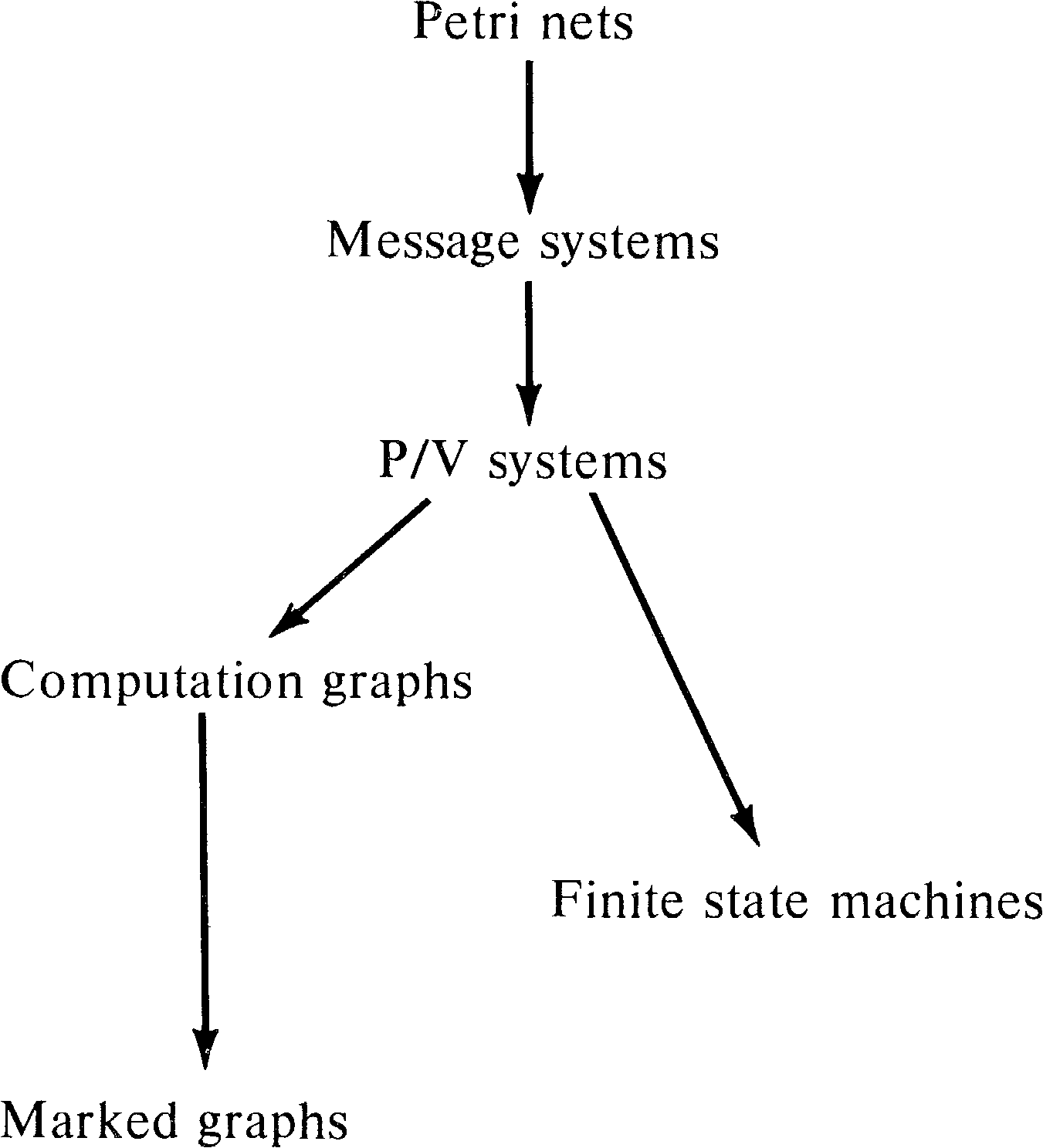Figure 8.13. Adding message systems to the hierarchy.

8.6. UCLA Graphs

Petri nets are a graph model of parallel computation. Another graph model has been developed at the University of California at Los Angeles under the direction of Professor Estrin. This model is the complex bilogic graph model of computation (or UCLA graph model) [Baer 1968; Baer et al. 1970; Volansky 1970; Gostelow 1971; Cerf 1972]. In this model, systems are represented by a graph with complex directed arcs. A complex arc is an arc with (potentially) multiple sources and destinations.

Combinational logic controls the sequencing of operations at the nodes. If the input logic of a node is AND (*), tokens are needed on each input arc to enable an operation. For OR (+) logic, tokens are only needed on some one input arc. Execution of the node removes the enabling tokens on the input arcs and places tokens on the output arcs according to the output logic. For AND output logic, tokens are placed on all output arcs, while for OR logic, tokens are placed on any one output arc. The number of tokens involved for a given node-arc pair is the degree (or multiplicity) of that pair and may be any nonnegative integer.

Figure 8.14 is an example UCLA graph. Notice that some arcs have multiple sources (tails) and destinations (heads). Also notice that the logic of each arc-node pair is marked on the graph as either * for AND logic or + for OR logic. The degree of an arc is indicated by a small number where the arc meets the node. The degree is omitted if it is one, as is the logic when only one arc is the input to a node. In the example node a can fire whenever arc S has a token. When node a fires, it removes the token from arc S and puts tokens on both arc A and arc B (AND logic). Node g, on the other hand, will place a token on either arc K or arc G (OR logic). Node i is enabled whenever there are two tokens on arc J or one token on arc K.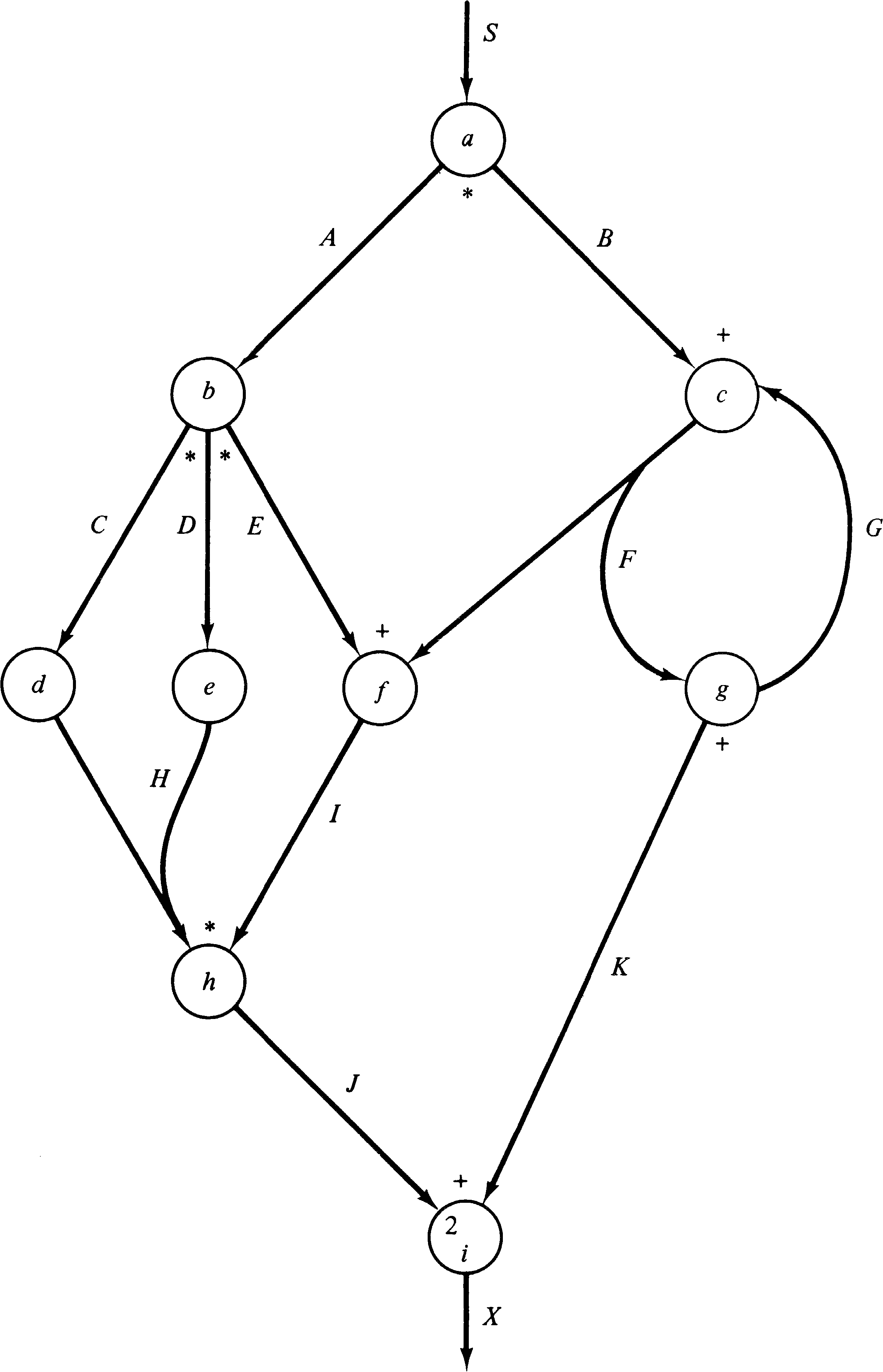Figure 8.14. A complex bilogic directed graph (UCLA graph).

DEFINITION 8.1
A UCLA graph is a six-tuple C = (V, A, L, Q, S, F), where

 V = { v1, v2, …, vr } is a set of vertices A = { a1, a2, …, as } is a set of arcs L = (L−, L+): V → { *, + } is the input(L−) and output(L+) logic mapping for each vertex Q = (Q−, Q+): V ⨯ A → N is the input(Q−) and output(Q+) degree of each arc−vertex pair S ∈ A is the start arc F ∈ A is the final arc

The arcs of the graph are defined as ordered pairs of sets of vertices. The first component of the pair is the set of input vertices, and the second component is the set of output vertices. The start arc has an empty set of input vertices, and the final arc has an empty set of output vertices.

The transformation from a UCLA graph to a Petri net is straightforward due to the similarity of the two systems. Every arc in a UCLA graph is represented by a place in a Petri net. In addition, we represent a node v by a place pv and two transitions tv′ and tv′′ . The first transition tv′ represents the initiation of the operation associated with node v; the second transition represents the termination of the operation. This is sketched in Figure 8.15. (The modeling of UCLA graph nodes by initiation and termination transitions is not strictly necessary but is convenient.) Figure 8.16 indicates how the input and output logic for UCLA graphs is represented in an equivalent Petri net. Degrees greater than 1 are modeled by multiple arcs between places and transitions in the Petri net.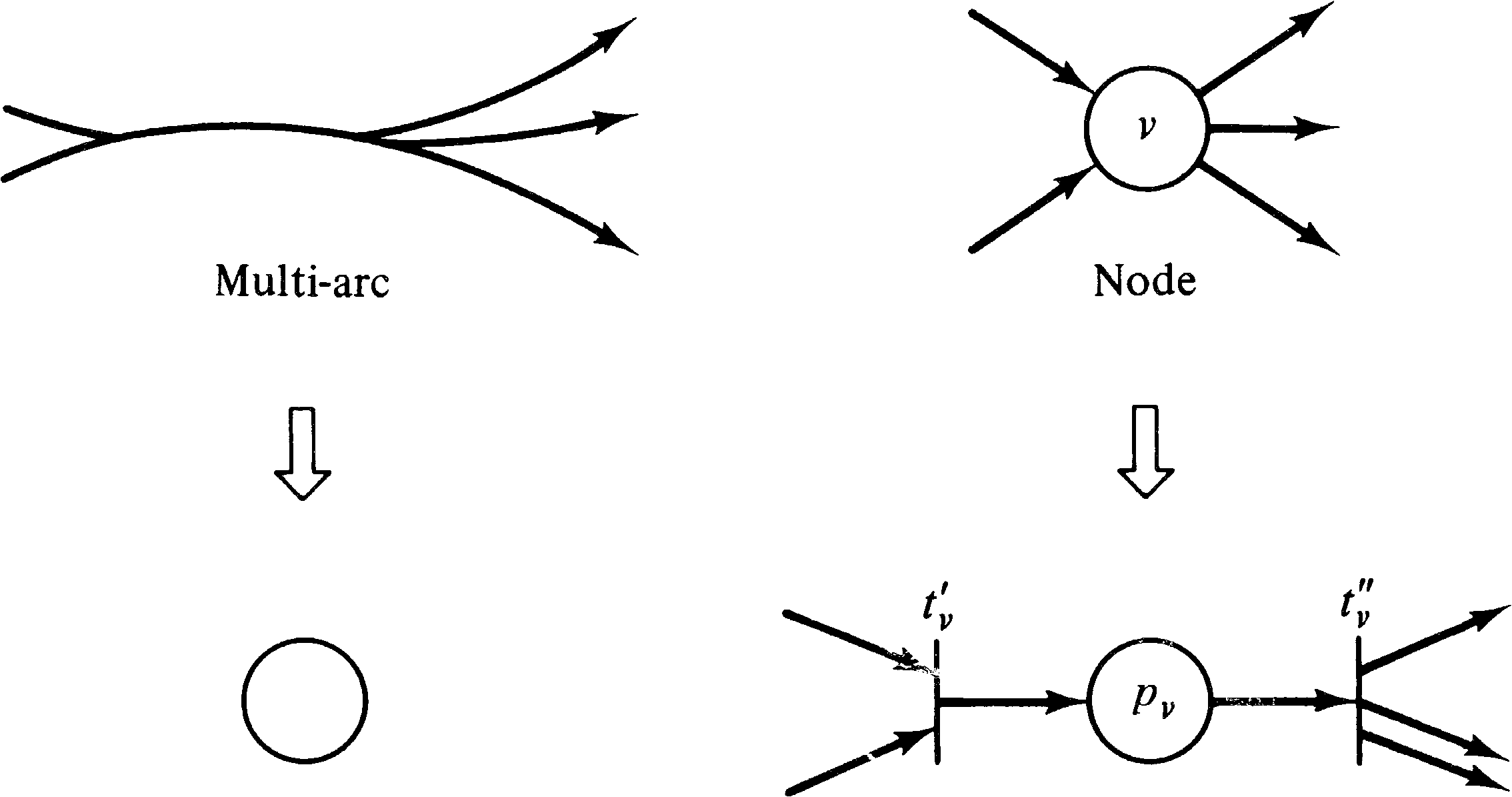Figure 8.15. Basic representation of the elements of a UCLA graph as a Petri net.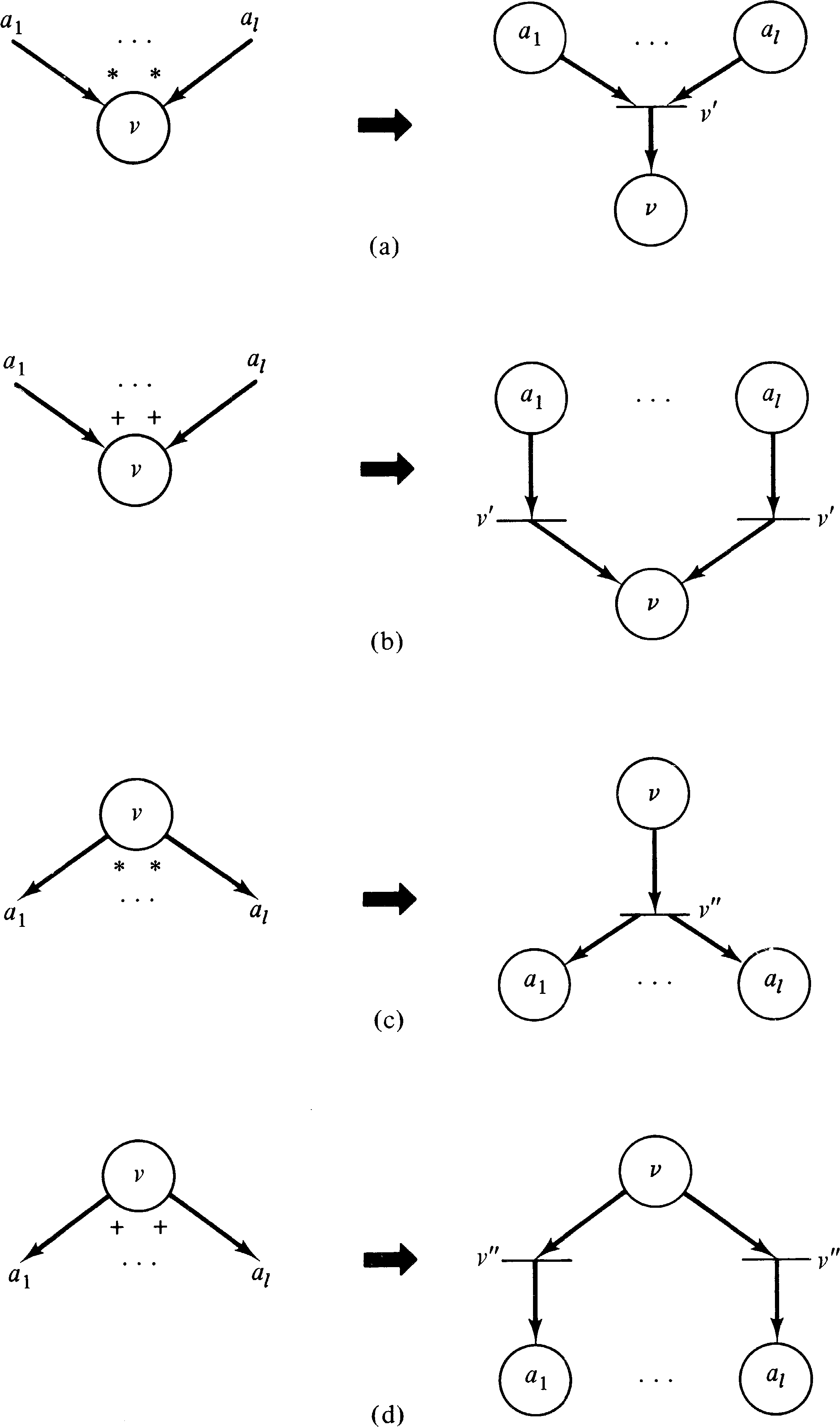Figure 8.16. Transformation of UCLA graph parts into Petri nets.

Figure 8.17 transforms the UCLA graph of Figure 8.14 into an equivalent Petri net.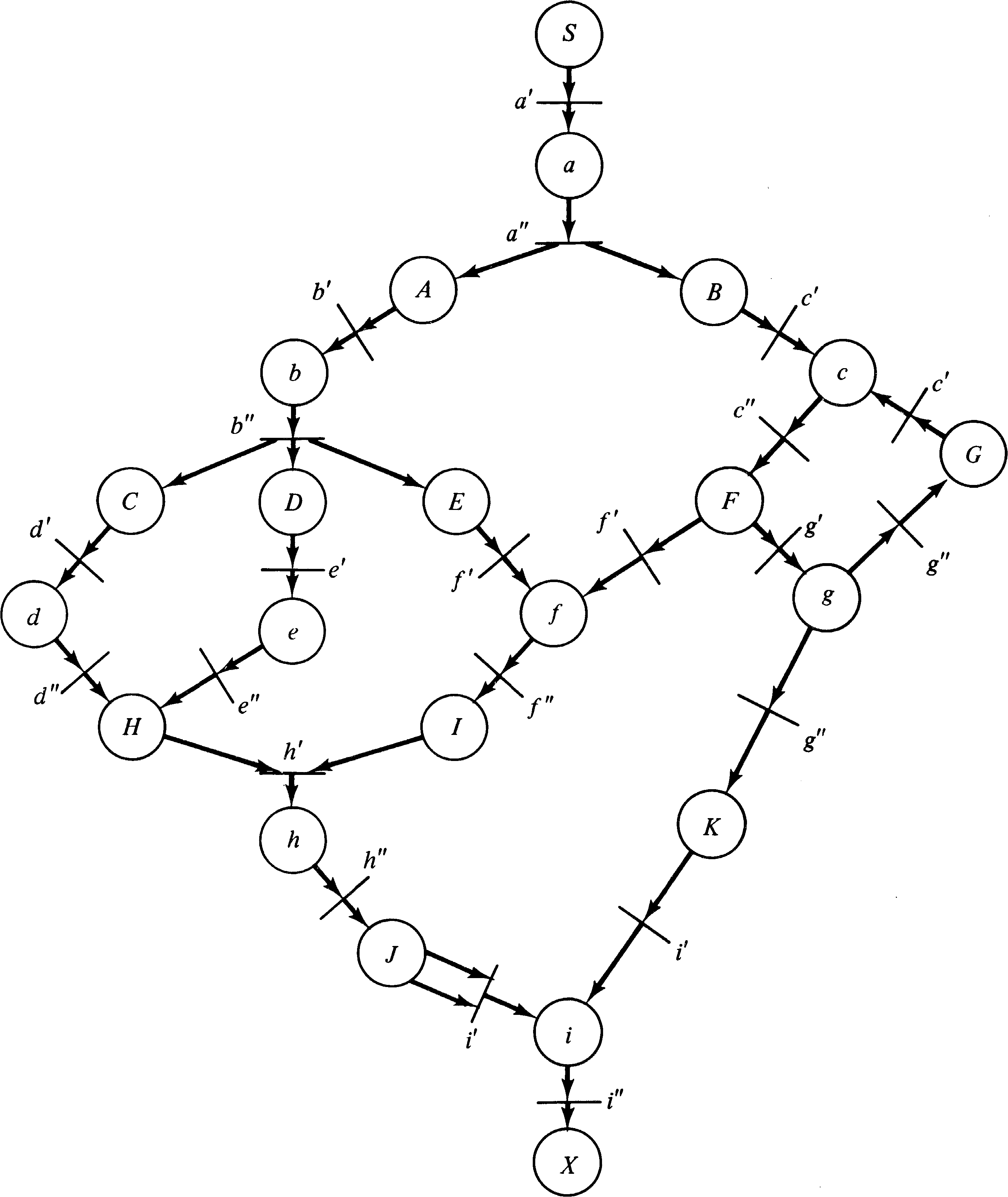Figure 8.17. The Petri net equivalent of the UCLA graph of Figure 8.14.

This transformation shows that the modeling power of UCLA graphs is included in the modeling power of Petri nets. It should be obvious that a Petri net can be converted into an equivalent UCLA graph by representing places as UCLA graph arcs and transitions as nodes with AND input and output logic. Thus these two models are equivalent in modeling power. Figure 8.18 shows the modified hierarchy of models.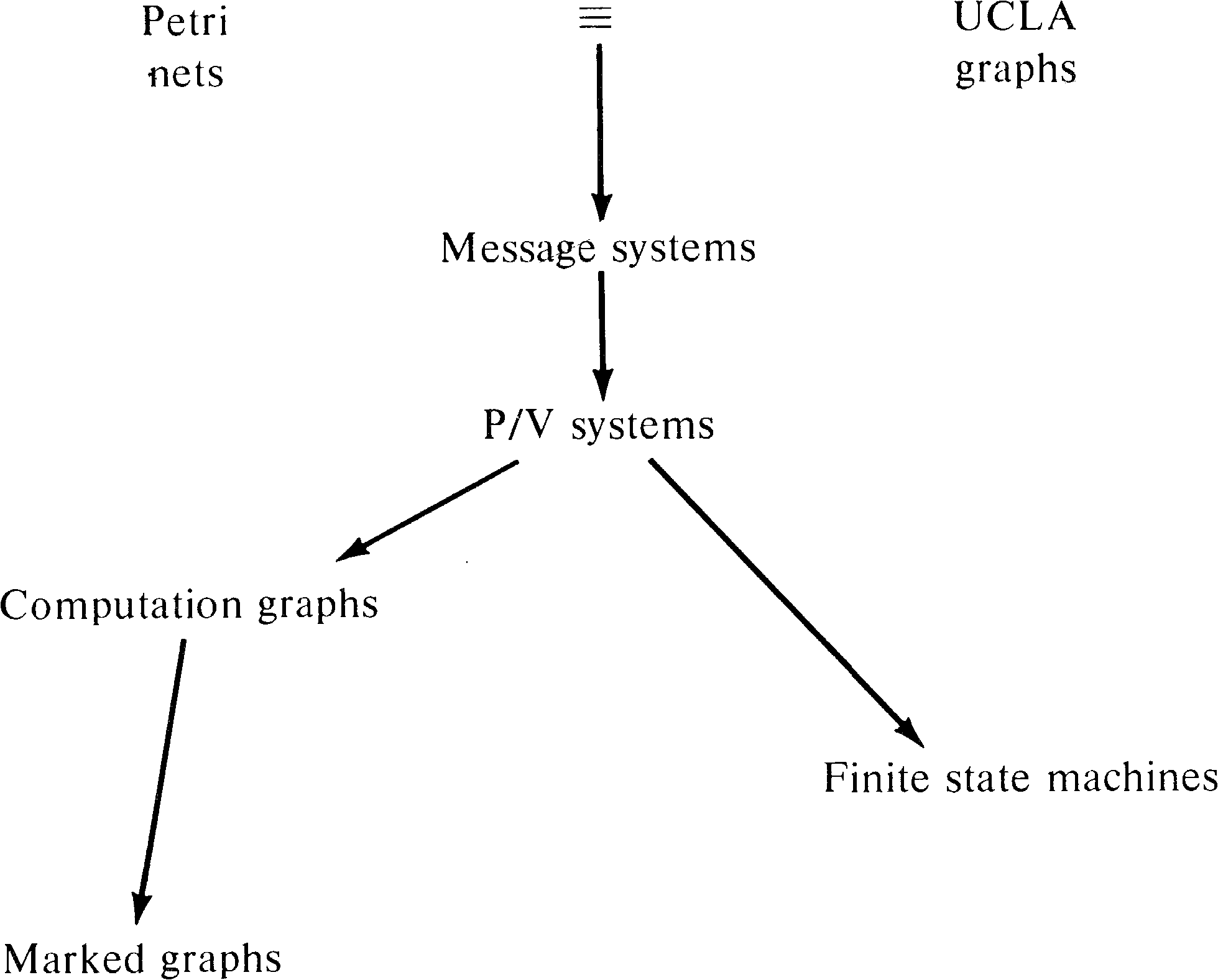Figure 8.18. Adding UCLA graphs to the hierarchy.

8.7. Vector Addition and Replacement Systems

If you have glanced at the bibliography you may have noticed that many of the references have titles referring not to Petri nets but to vector addition systems. Vector addition systems were introduced by Karp and Miller  as a mathematical tool for analyzing systems of parallel processes. Because of their simple mathematical formulation, vector addition systems are typically used for formal proofs of properties of Petri nets or similar systems.

DEFINITION 8.2
A vector addition system V is a pair V = ( B, s ), where B = { b1, b2, …, bm } is a set of m vectors, called basis or displacement vectors. The vector s is the start vector. All vectors are composed of n integer values. The elements of s are nonnegative.

The reachability set of a vector addition system V is denoted R ( V ) and can be defined either recursively by

DEFINITION 8.3
The reachability set R ( V ) for a vector addition system V = ( B, s ) is the smallest set such that

1. sR ( V ) .

2. If xR ( V ) and ( x + bj ) ≥ 0, then ( x + bj ) ∈ R ( V ) .
or by
DEFINITION 8.4
xR ( V ) if there exists a sequence bi1, bi2, …, bik of basis vectors such that

 k x = s + Σ bij j = 1 r s + Σ bij ≥ 0 for all r, 0 ≤ r ≤ k j = 1

With these definitions, it is easy to see that vector addition systems and Petri nets are equivalent. Given a Petri net, we can construct a vector addition system whose start vector s is the initial marking, with one basis vector for each transition. The n components of the vectors of the vector addition system correspond to the markings of the n places of the Petri net, or in the case of the basis vectors to the change in marking resulting from firing the associated transition.

Similarly, a vector addition system can be converted into an equivalent Petri net, using places for the components of the vectors and transitions to represent basis vectors. Figure 8.19 illustrates the equivalence of these two models.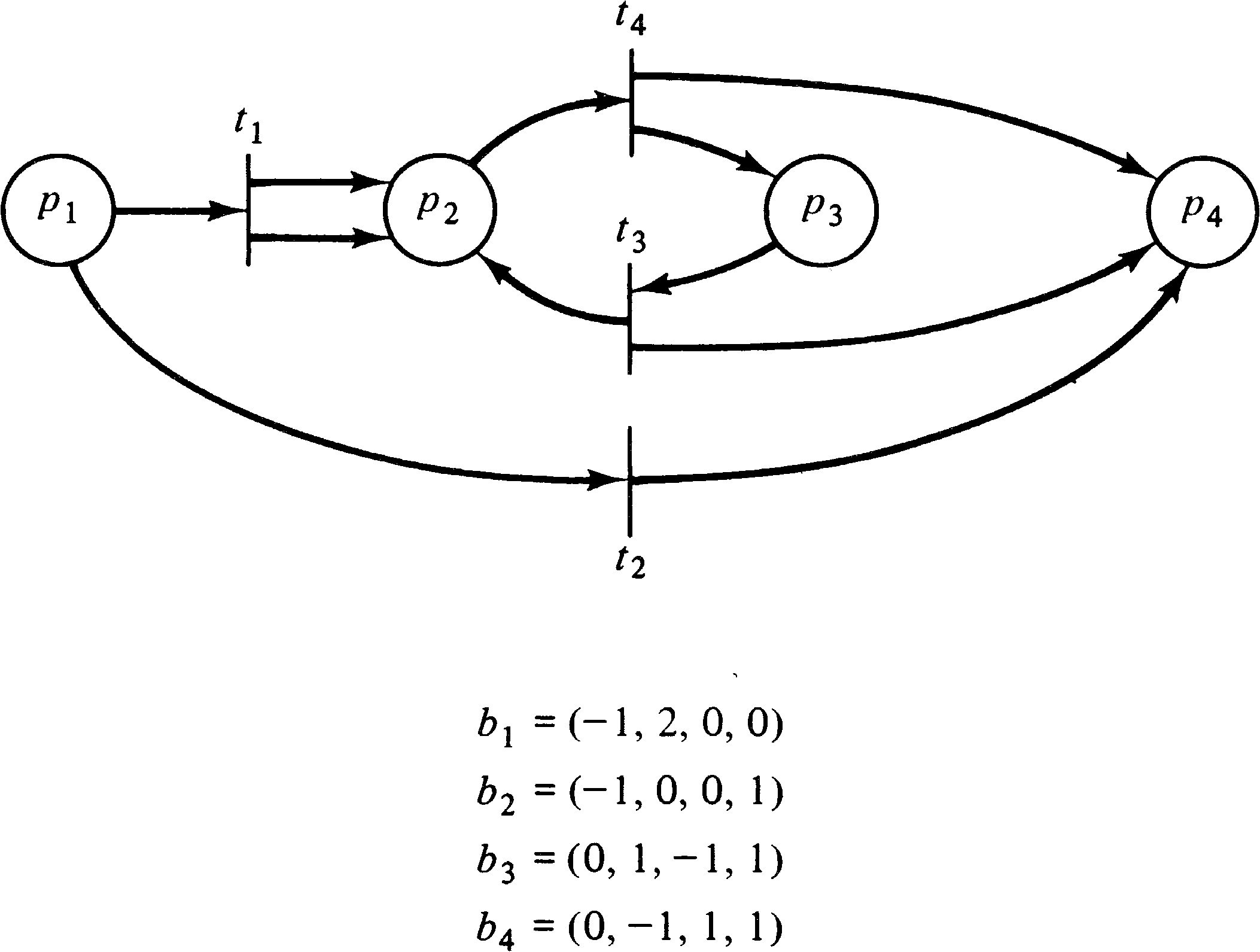Figure 8.19. A Petri net and its equivalent vector addition system.

In fact, vector addition systems are equivalent to self-loop-free Petri nets. Remember that with a self-loop, the change is zero, but the number of tokens in the self-loop place must be nonzero. This does not diminish the power of vector addition systems, since we have seen (in Section 5.3) that self-loop-free Petri nets are equivalent to general Petri nets. However, to more directly model Petri nets with self-loops in a vector addition system-like model, Keller has defined vector replacement systems [Keller 1972].

DEFINITION 8.5
A vector replacement system consists of a start vector s ≥ 0 and m pairs of vectors (Ui, Vi ) such that UiVi.

The Ui vectors are called test vectors. The reachability set is redefined such that s is in the reachability set, and if x is in the reachability set and x + Ui ≥ 0, then x + Vi is in the reachability set.

The vector replacement system model explicitly separates the test for enabling a transition from the action of firing the transition. The equivalence of vector replacement systems to (general) Petri nets is obvious.

Adding vector addition systems and vector replacement systems to our hierarchy yields Figure 8.20. The importance of vector addition and replacement systems stems from their concise mathematical definition and the usefulness of this definition in proofs of the mathematical properties of systems.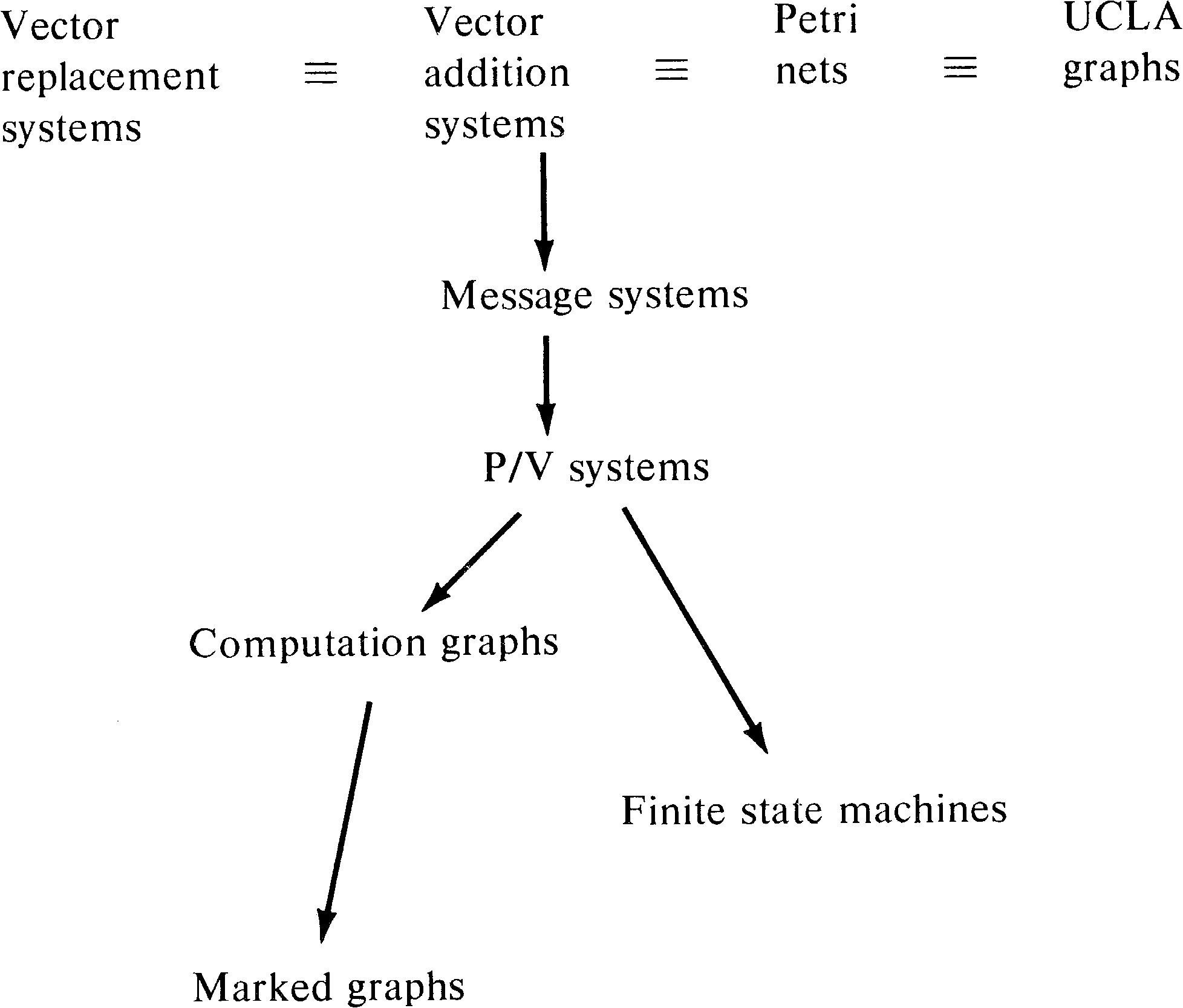Figure 8.20. Adding vector addition systems and vector replacement systems to the hierarchy.

8.8. Extended Petri Net Models

As a last addition to our hierarchy, we again mention the extended Petri net models studied in Chapter 7: Petri nets with constraints, exclusive-OR transitions, switches, inhibitor arcs, priorities, or times. We have seen that all of these models are equivalent to Turing machines. Thus these models properly include Petri net models. Our final hierarchy of models is shown in Figure 8.21.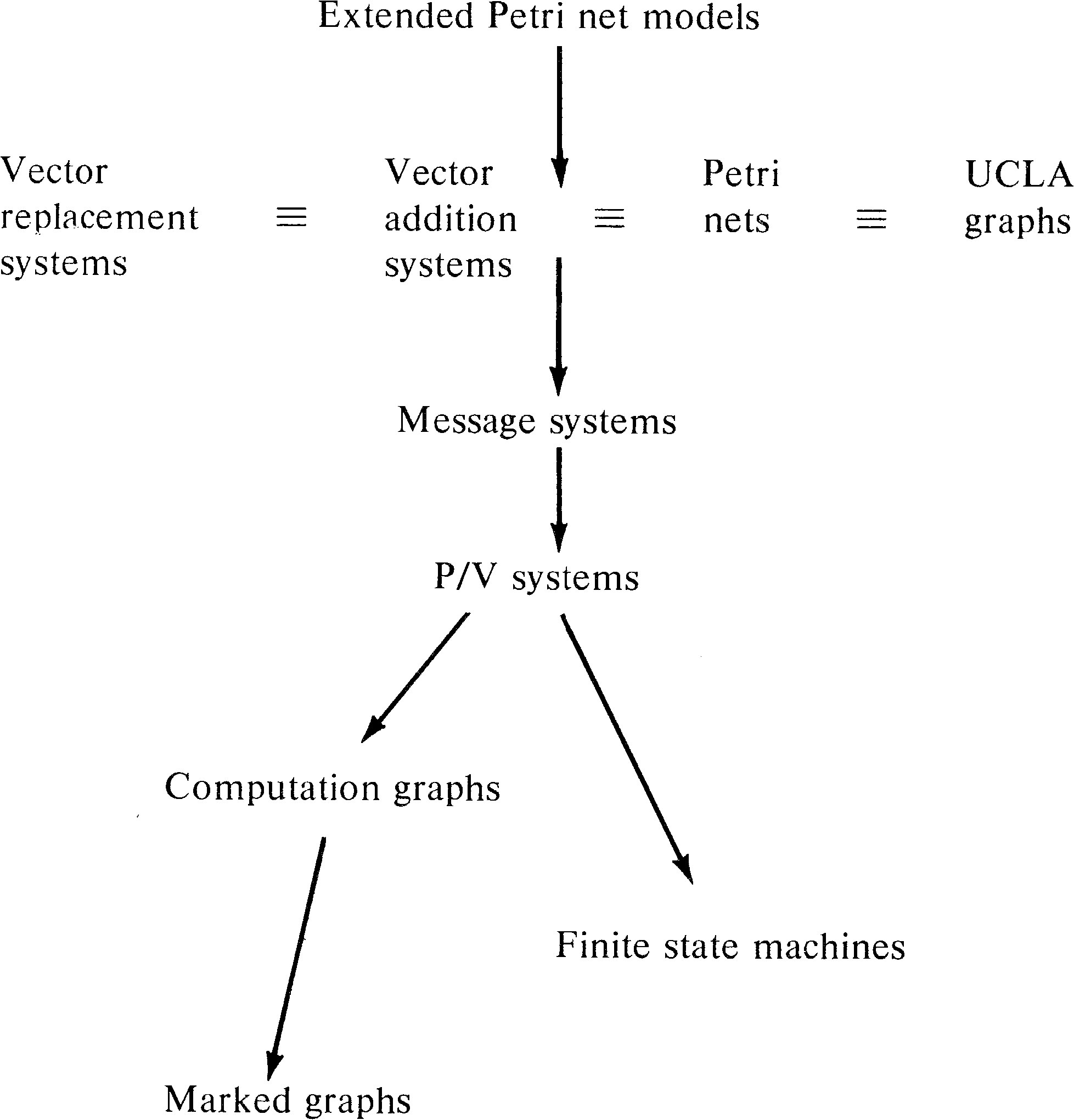Figure 8.21. The complete hierarchy of models of parallel computation.

The studies by Peterson and Bredt , Agerwala [1974b], and Lipton et al.  should be read first, since these are the most directly related works. Also read the surveys by Bredt [1970a] and Baer [1973a] and the work of Miller [1973; 1974]. The references in these papers will lead to the original work on the individual models.

Riddle's model [Riddle 1972] would appear to be the best for modeling large software systems and deserves detailed study.

8.10. Topics for Further Study

1. Expand the hierarchy of Figure 8.21 by including the restricted Petri net models discussed in Chapter 7: free-choice Petri nets and simple Petri nets.

2. Investigate the properties of the languages defined by the classes of models of this chapter and relate them to regular, context-free, and context-sensitive languages.

3. Determine the decidability of the reachability problem for each of the classes of models discussed in this chapter.

4. Extend the work of this chapter to include the models of Adams [1968; 1970], Luconi , Rodriguez , Seitz , and Slutz .